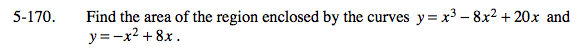### Home > CALC > Chapter 5 > Lesson 5.5.2 > Problem5-170

5-170.

Find the area of the region enclosed by the curves y = x3 − 8x2 + 20x and y = −x2 + 8x. Homework Help ✎Find the points in which the curves intersect. There will be THREE!

Since there are three points of intersections, there might be two integrals that need to be evaluated.

For each integral, determine which function is on top and which is on the bottom. (You could do this by evaluating points between the bounds OR by sketching the functions.)

Integrate.